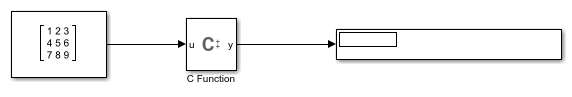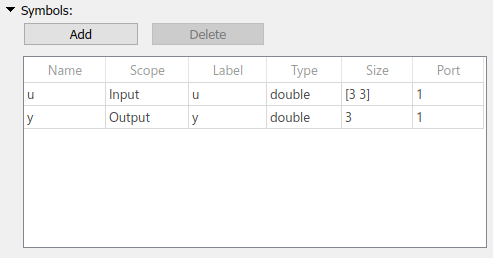# Access Elements of a Matrix Using Output Code in a C Function Block

This example shows how to access elements of a matrix from a C Function block using the Output Code pane.

```open_system('mMatrixColumnSumOutputCode') ```Elements in each column of the input matrix are accessed and added to calculate a sum for each column.You must define all symbols used in code on the Symbols table of the Block Parameters. To set the size of the input matrix, specify size as `[r c]`. `r` corresponds to the number of rows in the input matrix and `c` corresponds to the number of columns in the input matrix.